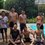# Utomo Theorem ~ The Next Millenium Prize Problem

Utomo Theorem For every prime numbers p which p + 2 is also prime, then $2^{p+2}$ - 1 always prime.

Can you proves this conjecture?Note by Budi Utomo
4 years ago

This discussion board is a place to discuss our Daily Challenges and the math and science related to those challenges. Explanations are more than just a solution — they should explain the steps and thinking strategies that you used to obtain the solution. Comments should further the discussion of math and science.

When posting on Brilliant:

• Use the emojis to react to an explanation, whether you're congratulating a job well done , or just really confused .
• Ask specific questions about the challenge or the steps in somebody's explanation. Well-posed questions can add a lot to the discussion, but posting "I don't understand!" doesn't help anyone.
• Try to contribute something new to the discussion, whether it is an extension, generalization or other idea related to the challenge.
• Stay on topic — we're all here to learn more about math and science, not to hear about your favorite get-rich-quick scheme or current world events.

MarkdownAppears as
*italics* or _italics_ italics
**bold** or __bold__ bold
- bulleted- list
• bulleted
• list
1. numbered2. list
1. numbered
2. list
Note: you must add a full line of space before and after lists for them to show up correctly
paragraph 1paragraph 2

paragraph 1

paragraph 2

[example link](https://brilliant.org)example link
> This is a quote
This is a quote
    # I indented these lines
# 4 spaces, and now they show
# up as a code block.

print "hello world"
# I indented these lines
# 4 spaces, and now they show
# up as a code block.

print "hello world"
MathAppears as
Remember to wrap math in $$ ... $$ or $ ... $ to ensure proper formatting.
2 \times 3 $2 \times 3$
2^{34} $2^{34}$
a_{i-1} $a_{i-1}$
\frac{2}{3} $\frac{2}{3}$
\sqrt{2} $\sqrt{2}$
\sum_{i=1}^3 $\sum_{i=1}^3$
\sin \theta $\sin \theta$
\boxed{123} $\boxed{123}$

## Comments

Sort by:

Top Newest

What you mean is the larger one of every twin prime would produce a Mersenne Prime ? Oh I need to check this out

Log in to reply

It's actually easier to list the primes $p$ which satisfy your statement than to find the list that fails it. Just compare any list of twin primes with a list of Mersenne primes, and you'll see that the $p$ which satisfy your statement are $p = 3, 5, 11, 17, 29, 59, 1277, 4421, 110501, 132047, \ldots$ and at this point, I got a little tired of looking.

Point being, in the first 8000 or so twin primes, only these 10 satisfy your statement, so there's no way to patch it by removing just a few errant counterexamples.

- 4 years ago

Log in to reply

maybe, except just for p = 41.

- 4 years ago

Log in to reply

you sure that 2^43 - 1 isn't prime number :(

- 4 years ago

Log in to reply

but, in f(p) = 2^p - 1 hasn't always gives a prime marsenne.

- 4 years ago

Log in to reply

From this theorem, we knew that a large primes is infinite.

- 4 years ago

Log in to reply

For a counter example, take p=41, 2^43-1 is not prime. See this

Log in to reply

Actually, the numbers of the form 2^n-1 that are prime are known as Mersenne primes. Read this

Log in to reply

×

Problem Loading...

Note Loading...

Set Loading...